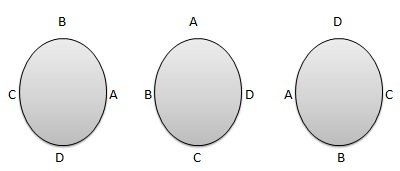# Statistics - Circular Permutation

Circular permutation is the total number of ways in which n distinct objects can be arranged around a fix circle. It is of two types.

• Case 1 − Clockwise and Anticlockwise orders are different.

• Case 2 − Clockwise and Anticlockwise orders are same.## Case 1 − Formula

${P_n = (n-1)!}$

Where −

• ${P_n}$ = represents circular permutation

• ${n}$ = Number of objects

## Case 2 − Formula

${P_n = \frac{n-1!}{2!}}$

Where −

• ${P_n}$ = represents circular permutation

• ${n}$ = Number of objects

## Example

### Problem Statement

Calculate circular permulation of 4 persons sitting around a round table considering i) Clockwise and Anticlockwise orders as different and ii) Clockwise and Anticlockwise orders as same.

## Solution

In Case 1, n = 4, Using formula

${P_n = (n-1)!}$

Apply the formula

${P_4 = (4-1)! \\[7pt] \ = 3! \\[7pt] \ = 6 }$

In Case 2, n = 4, Using formula

${P_n = \frac{n-1!}{2!}}$

Apply the formula

${P_4 = \frac{n-1!}{2!} \\[7pt] \ = \frac{4-1!}{2!} \\[7pt] \ = \frac{3!}{2!} \\[7pt] \ = \frac{6}{2} \\[7pt] \ = 3 }$